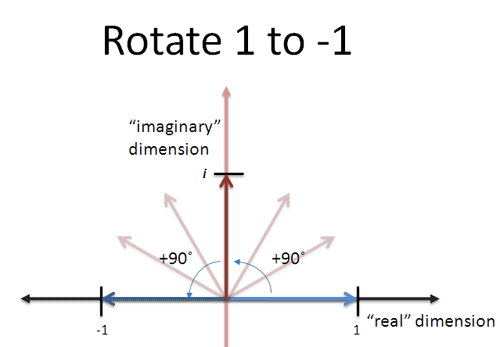# Making of a good explanation

Quick tips on building a “BetterExplained-style” explanation.

## 1. Find a short analogy

What is the simplest distillation of the aha! moment? Examples: “Imaginary numbers are rotations” or “e is continuously compounded growth”.

## 2. Make a quick diagram

Can the analogy be visualized?## 3. Work through an example based on the analogy

This could be a practice problem, or explaining the formal definition using the analogy.

If $i$ is a 90-degree rotation, then $i \cdot i$ should be a 180-degree rotation. And yes, $i^2 = -1$ . Similarly, $i^4$ should be a full rotation, or 1.

We can also work through a rotation problem…

Analogy:
Diagram:
Example:
Principle (plain English):
Theory (formal description)

Imaginary Numbers
Fourier Transform
Euler’s Formula

Can go recursive as well (for one subelement of the idea, get the analogy, diagram, example).

Note: the analogy depth can be adjusted for your level. For example, can compare Vector Calculus to earlier Calculus concepts. Use the existing knowledge of your audience when possible.

1 Like